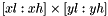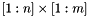# util::Aggreg2Dto1D< T, Pr, row > Class Template Reference

1D representation of a section of a prefix sum array by summing its element row-wise or column-wise. More...

#include <util/prefix_sum_tools.hpp>

## Detailed Description

### template<typename T, typename Pr, bool row> class util::Aggreg2Dto1D< T, Pr, row >

1D representation of a section of a prefix sum array by summing its element row-wise or column-wise.

This is a 1d prefix sum array with both the bracket[] notation defined by util::PrefixSumArray1DOfTBracket and interval notation defined by util::PrefixSumArray1DOfTBracket.

Parameters:
 T type of the array Pr type of the real array row select the orientation of the summation. (e.g. If row is true and the rectangle is of size 10x20, then the equivalent 1darray is of size 10)

List of all members.

## Public Member Functions

Aggreg2Dto1D (const Pr &p, int xl, int xh, int yl, int yh)
Reduces the 2D matrix into 1D array.
Aggreg2Dto1D (const Aggreg2Dto1D< T, Pr, row > &o)
const T operator[] (int x) const
T interval (int i1, int i2) const
Returns the load in a given interval.

## Private Member Functions

Aggreg2Dto1D< T, Pr, row > & operator= (const Aggreg2Dto1D< T, Pr, row > &rhs)
deactivated since it does not make sense
Aggreg2Dto1D ()
deactivated since it does not make sense

## Private Attributes

const Prpr
the 2d prefix sum array which is represented
const int xlow
const int xhigh
const int ylow
const int yhigh
T precomp

## Constructor & Destructor Documentation

template<typename T, typename Pr, bool row>
 util::Aggreg2Dto1D< T, Pr, row >::Aggreg2Dto1D ( )  [private]

deactivated since it does not make sense

template<typename T , typename Pr , bool row>
 util::Aggreg2Dto1D< T, Pr, row >::Aggreg2Dto1D ( const Pr & p, int xl, int xh, int yl, int yh )

Reduces the 2D matrix into 1D array.

Reduces the 2D matrix into 1D arrayin the original array(where n and m are last elements not sizes).

If row is true, there are (xh-xl) elements in the array.

Parameters:
 p prefixSumArray xl xlow of submatrix xh xhigh of submatrix yl ylow of submatrix yh yhigh of submatrix
template<typename T , typename Pr , bool row>
 util::Aggreg2Dto1D< T, Pr, row >::Aggreg2Dto1D ( const Aggreg2Dto1D< T, Pr, row > & o )

Copy constructor for Aggreg2Dto1D

## Member Function Documentation

template<typename T , typename Pr , bool row>
 T util::Aggreg2Dto1D< T, Pr, row >::interval ( int i1, int i2 ) const

Returns the load in a given interval.

The interval is ]i1,i2] i.e., i1 is excluded, i2 is included.

Parameters:
 i1 lower interval point i2 higher interval point
Returns:
template<typename T, typename Pr, bool row>
 Aggreg2Dto1D& util::Aggreg2Dto1D< T, Pr, row >::operator= ( const Aggreg2Dto1D< T, Pr, row > & rhs )  [private]

deactivated since it does not make sense

template<typename T , typename Pr , bool row>
 const T util::Aggreg2Dto1D< T, Pr, row >::operator[] ( int x ) const

## Member Data Documentation

template<typename T, typename Pr, bool row>
 const Pr& util::Aggreg2Dto1D< T, Pr, row >::pr [private]

the 2d prefix sum array which is represented

template<typename T, typename Pr, bool row>
 T util::Aggreg2Dto1D< T, Pr, row >::precomp [private]
template<typename T, typename Pr, bool row>
 const int util::Aggreg2Dto1D< T, Pr, row >::xhigh [private]
template<typename T, typename Pr, bool row>
 const int util::Aggreg2Dto1D< T, Pr, row >::xlow [private]
template<typename T, typename Pr, bool row>
 const int util::Aggreg2Dto1D< T, Pr, row >::yhigh [private]
template<typename T, typename Pr, bool row>
 const int util::Aggreg2Dto1D< T, Pr, row >::ylow [private]

The documentation for this class was generated from the following files: